# 神经网络中的Heloo，World，基于MINST数据集的LeNet# 1.MINST数据集

MNIST 数据集来自美国国家标准与技术研究所, National Institute of Standards and Technology (NIST). 训练集 (training set) 由来自 250 个人手写的数字构成, 其中 50% 是高中学生, 50% 来自人口普查局 (the Census Bureau) 的工作人员. 测试集(test set) 也是同样比例的手写数字数据.

MINST手写字体识别，大概相当于机器学习领域的”Hello World”的存在

### 1.1数据集的下载即载入

#### 1.2可以去官网下载MNIST handwritten digit database, Yann LeCun, Corinna Cortes and Chris Burges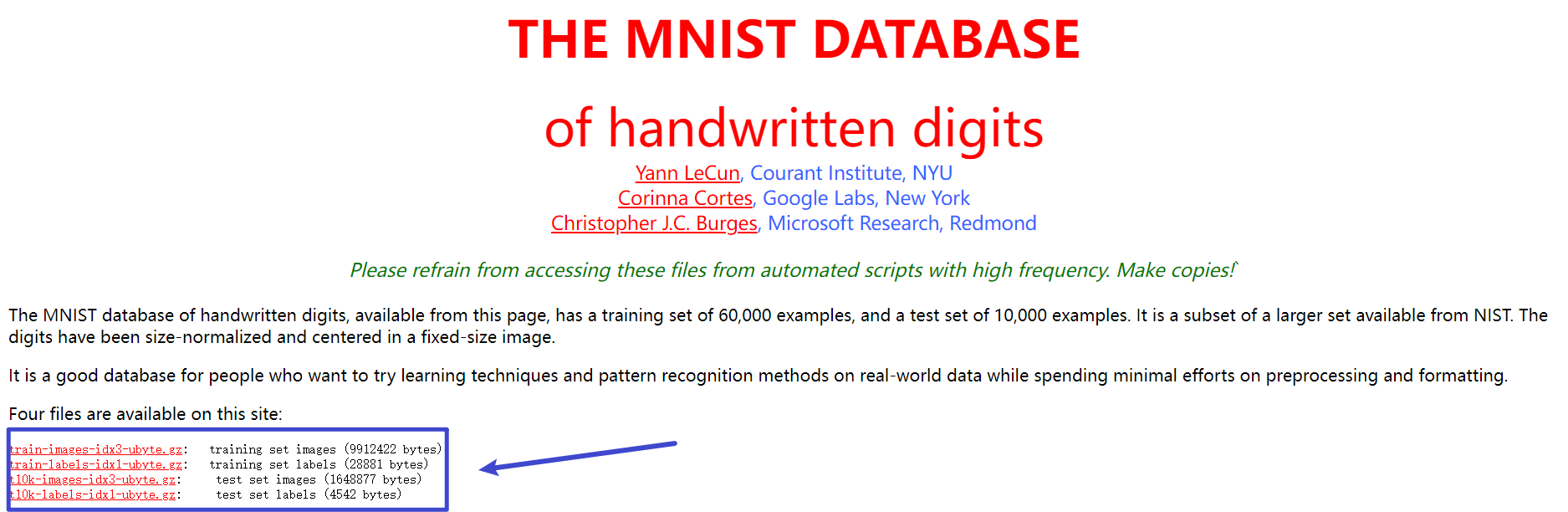``````# 设置训练数据集
data_train = torchvision.datasets.MNIST(
root='./data',
train=True,
transform=torchvision.transforms.ToTensor(),
``````
##### 2.2.1torchvision.datasets

torchvision.datasets是pytorch中集成的可以加载多个数据集的工具，包含图片分类，语义分割，视频等数据集，详情见

[Datasets — Torchvision 0.12 documentation (pytorch.org)](https://pytorch.org/vision/stable/datasets.html?highlight=utils data dataloader)进入该页面我们找到MINST就可以查看到使用MINST所需要的参数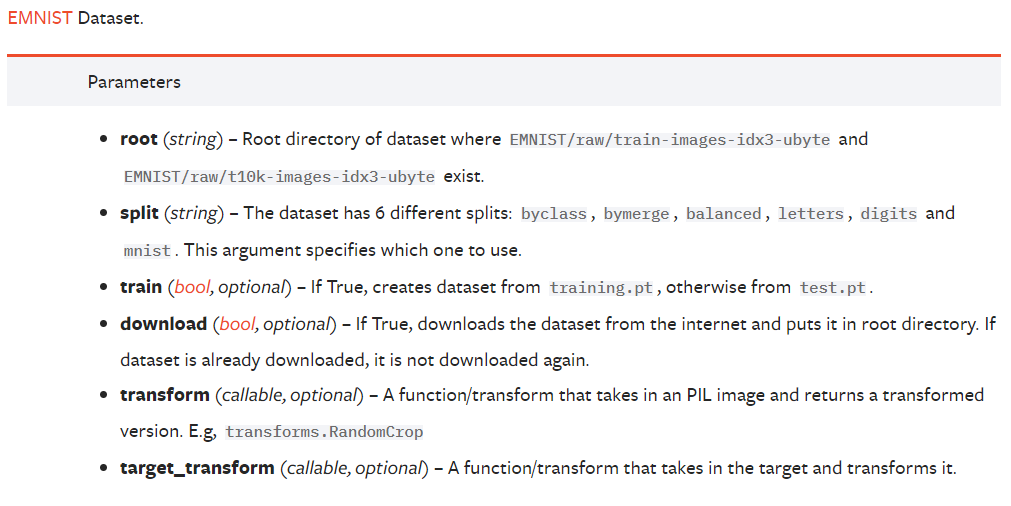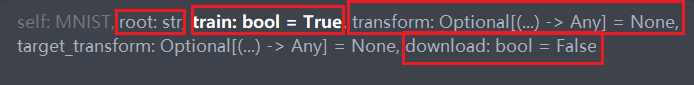4.transform在pytorch封装了许多图像处理的方法，如填充(Pad)，Resize，CenterCrop(中心裁剪)，Grayscale(灰度图)，更多方法见：Illustration of transforms — Torchvision 0.12 documentation (pytorch.org)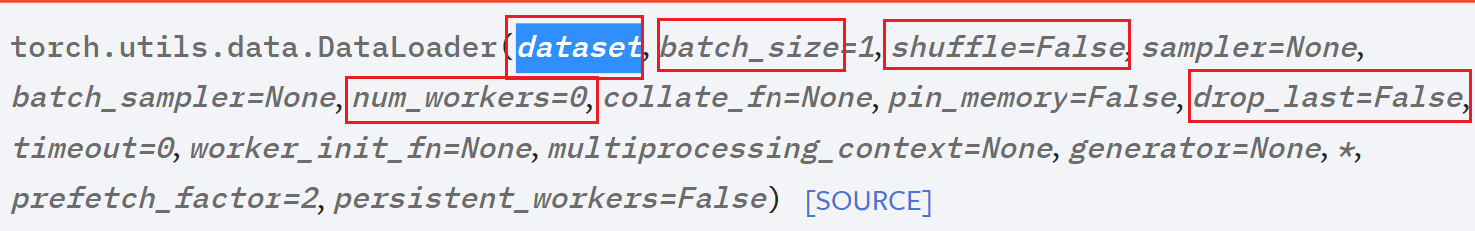3.MINST的内容的展示

(22条消息) MNIST数据集转为图片形式输出_zzZ_CMing的博客-CSDN博客_mnist图片 这篇博客有写怎么将MINST的数据保存到本地

``````#enumerate() 函数用于将一个可遍历的数据对象(如列表、元组或字符串)组合为一个索引序列，同时列出数据和数据下标
#next() 返回迭代器的下一个项目。
batch_idx, (example_data, example_targets) = next(examples)
# 绘制查看数据集内容
fig = plt.figure()
for i in range(16):
plt.subplot(4,4,i+1)  #设置图像显示为2行3列
plt.tight_layout()  #tight_layout会自动调整子图参数，使之填充整个图像区域。
plt.imshow(example_data[i], cmap='gray')
plt.title("Truth Number: {}".format(example_targets[i]))
plt.xticks([])    #不显示横纵坐标
plt.yticks([])
plt.show()
``````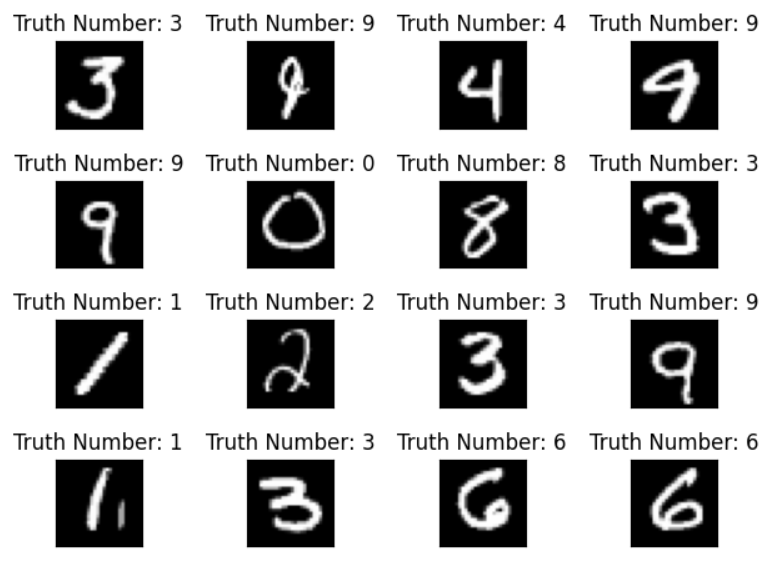debug一下，发现图像的尺寸为[1000,1,28,28]，分别代表batch_size为1000，chanel(通道)数为1(灰度图)，图像尺寸为28×28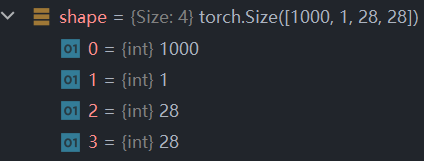# 2. MINST的训练和测试方法

### 2.1定义训练方法

``````def train(net, epoch=1):
net.train()#设置training mode
run_loss = 0#损失
for num_epoch in range(epoch):	#设置训练的轮数为epoch轮
print(f'epoch {num_epoch}')
#            x, y = data, data	#也可以这么写
x,y = data	#x,y分别代表数据和标注
outputs = net(x) #将数据放入神经网络中
loss = criterion(outputs, y) #计算损失
loss.backward()	#反向传播
optimizer.step() #优化器更新参数
run_loss += loss.item()
if i % 100 == 99:	#每训练100轮打印一次
print(f'[{(i + 1) * 100} / 60000] loss={run_loss / 100}')
run_loss = 0
test(net)
``````

#### net.train()的作用如下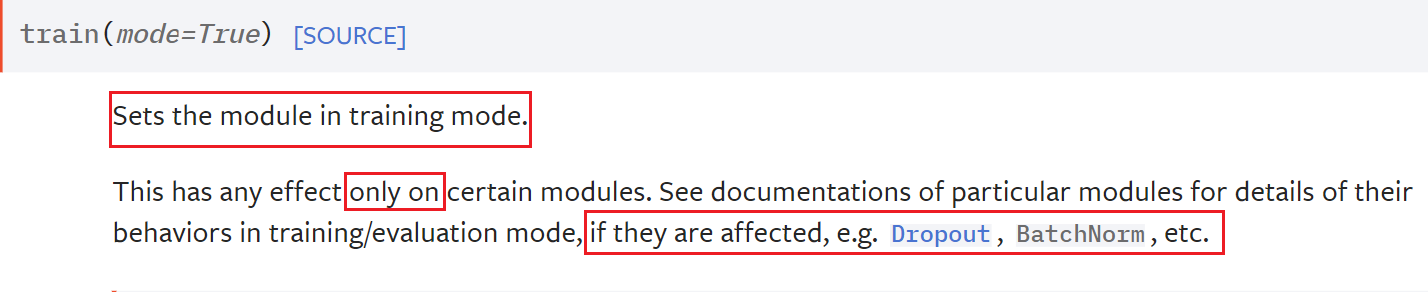#### 优化器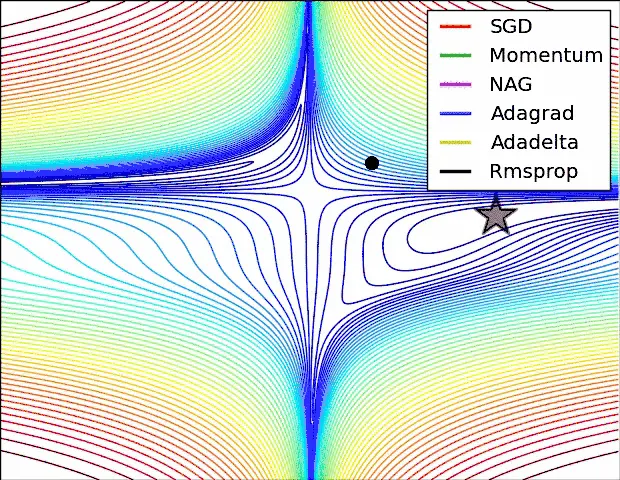### 2.2定义测试方法

``````def test(net):
net.eval()#和上面的net.train()一样
#        x, y = test_data, test_data
x,y = data
outputs = net(x)
pred = torch.max(outputs, 1)
print(f'test acc: {sum(pred == y) / outputs.shape}')#计算出损失(每个之和除以数量)
``````

# 3.定义神经网络

### 3.1 神经网络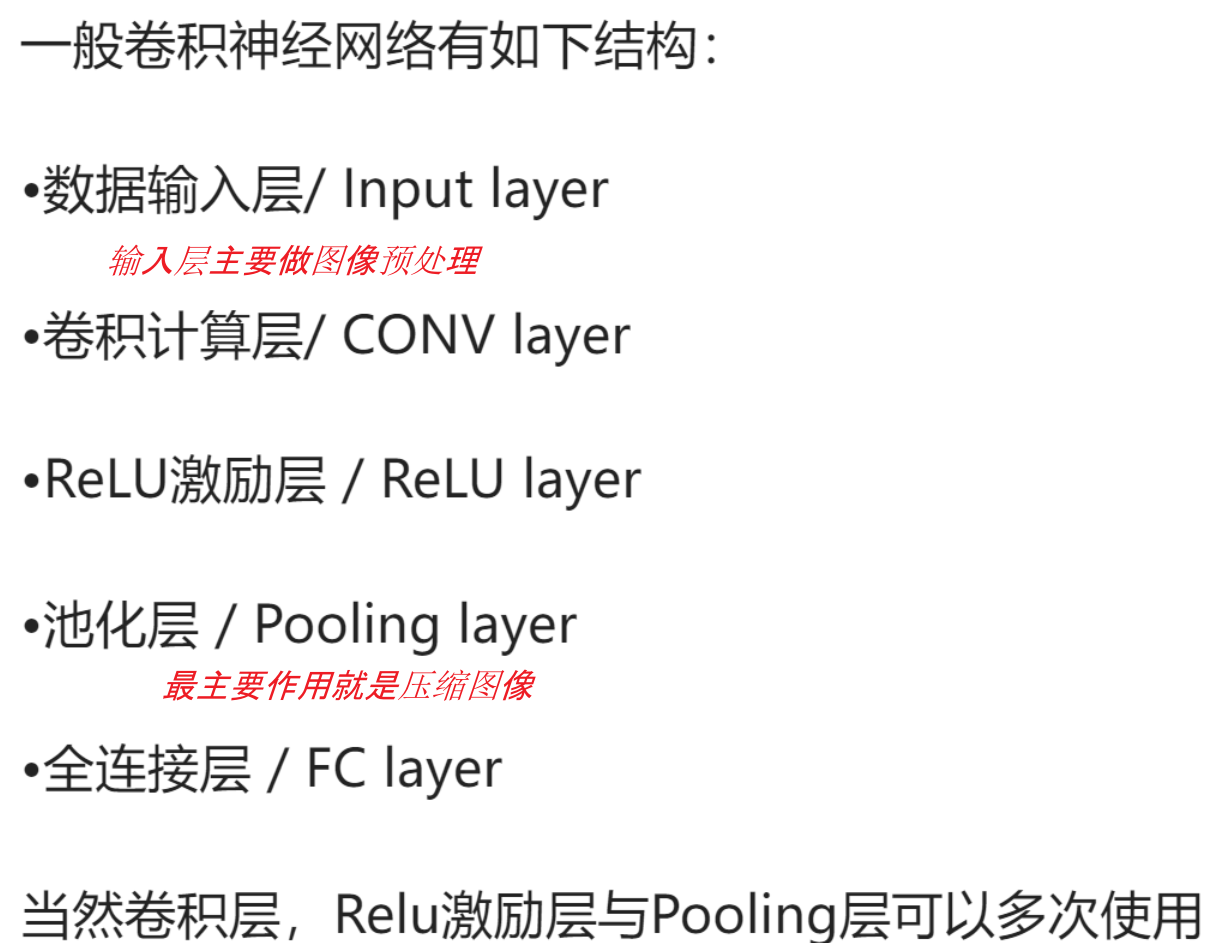#### 3.1.1. 卷积层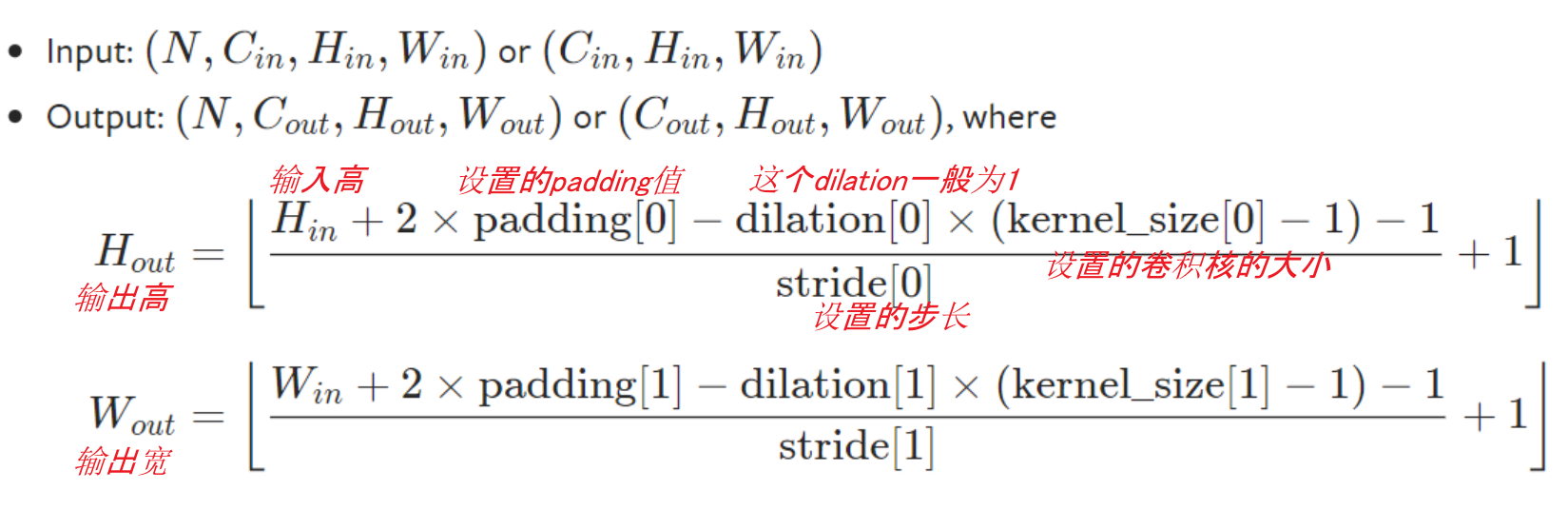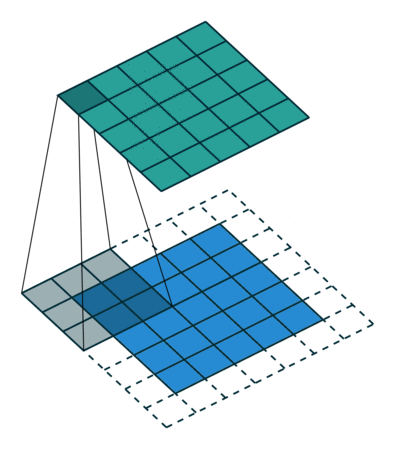#### 3.1.2. 池化层#### 3.1.3. 激励层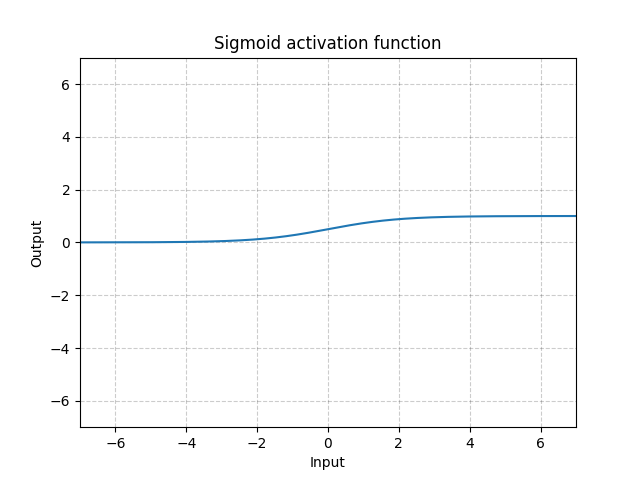### 3.2 LeNet

LeNet 是几种神经网络的统称，它们是 Yann LeCun 等人在 1990 年代开发的。一般认为，它们是最早的卷积神经网络（Convolutional Neural Networks, CNNs）。模型接收灰度图像，并输出其中包含的手写数字。LeNet 包含了以下三个模型：

• LeNet-1：5 层模型，一个简单的 CNN。
• LeNet-4：6 层模型，是 LeNet-1 的改进版本。
• LeNet-5：7 层模型，最著名的版本。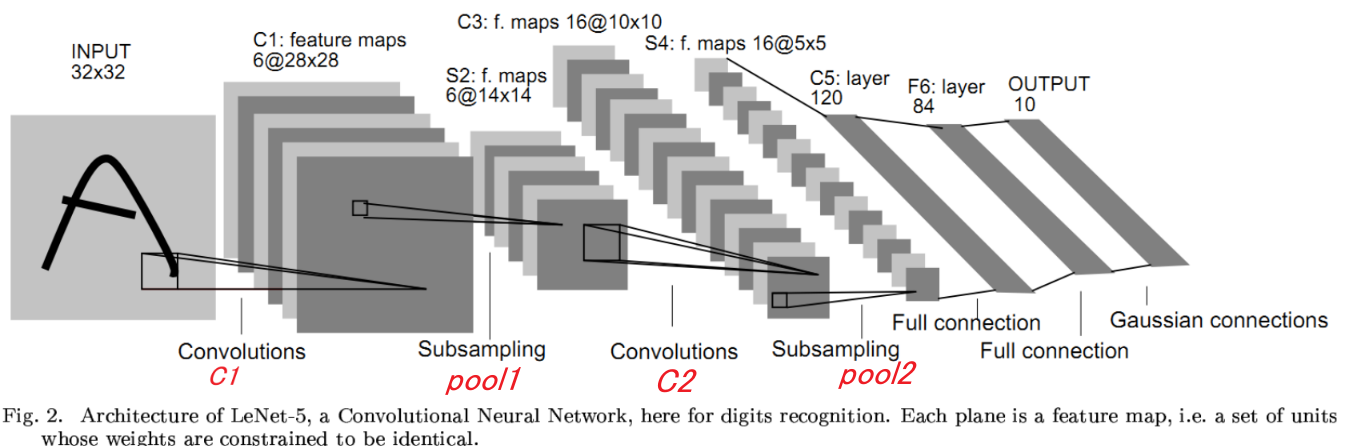``````class LeNet5(nn.Module):
def __init__(self):
super().__init__()
#这个注释的比较详细
self.conv1 = nn.Conv2d(in_channels=1, out_channels=6, kernel_size=5, padding=2)
# self.pool1 = nn.AvgPool2d(2) #本来LeNet使用的平均池化不如最大池化
self.pool1 = nn.MaxPool2d(kernel_size=2)
self.pool1 = nn.MaxPool2d(2)
self.conv2 = nn.Conv2d(6, 16, 5)
# self.pool2 = nn.AvgPool2d(2)
self.pool2 = nn.MaxPool2d(2)
self.fc1 = nn.Linear(16 * 5 * 5, 120)
self.fc2 = nn.Linear(120, 84)
self.fc3 = nn.Linear(84, 10)
#前向传播
def forward(self, x):
# x = torch.tanh(self.conv1(x)) #tanh在这里不如relu
x = torch.relu(self.conv1(x))
x = self.pool1(x)
# x = torch.tanh(self.conv2(x))
x = torch.relu(self.conv2(x))
x = self.pool2(x)

x = x.view(-1, 16 * 5 * 5)	#改变矩阵维度
x = self.fc1(x)
x = self.fc2(x)
x = self.fc3(x)
return x
``````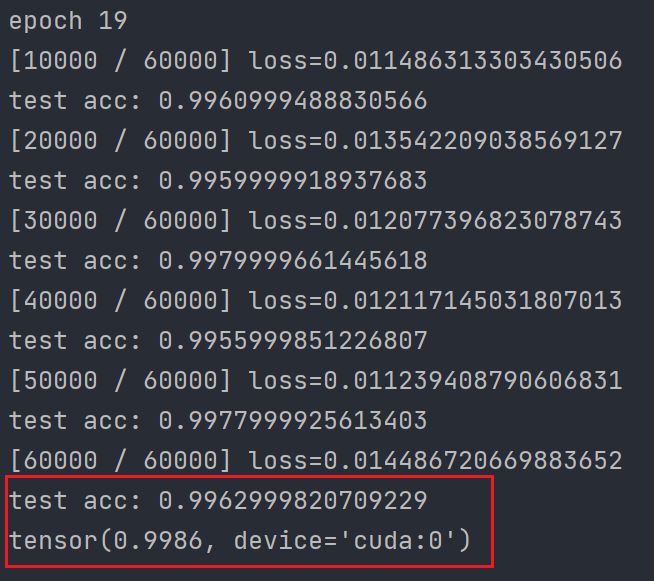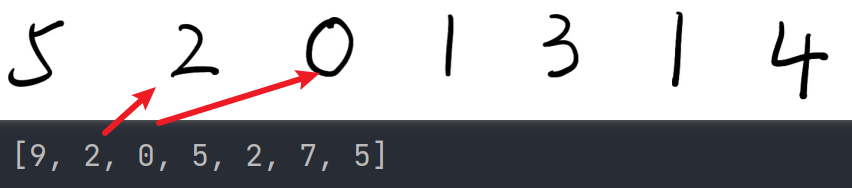# 4.完整代码

``````import torch
import torchvision
import torch.nn as nn
import torchvision.transforms as transforms
from torch.nn import ReLU
import torch.nn.functional as F
import numpy as np
import matplotlib.pyplot as plt
from torch.optim import optimizer

from PIL import Image
# 用来记录最佳的acc
best_acc = 0

trans_to_tensor = transforms.Compose([
transforms.ToTensor()
])

data_train = torchvision.datasets.MNIST(
root='./data',
train=True,
transform=torchvision.transforms.ToTensor(),

data_test = torchvision.datasets.MNIST(
'./data',
train=False,
transform=trans_to_tensor,

#MINST的通用训练方法
def test(net):
global best_acc
net.eval()
# x, y = test_data, test_data
# x = x.cuda()
# y = y.cuda()
x, y = test_data
outputs = net(x)
pred = torch.max(outputs, 1)
acc = sum(pred == y) / outputs.shape
if acc >  best_acc:
best_acc = acc
print(f'test acc: {acc}')
# net.train()

def train(net, epoch=1):
net.train()
run_loss = 0
for num_epoch in range(epoch):
print(f'epoch {num_epoch}')
# x, y = data, data
x,y = data
# print(data)
outputs = net(x)
loss = criterion(outputs, y)
loss.backward()
optimizer.step()
run_loss += loss.item()
if i % 100 == 99:
print(f'[{(i + 1) * 100} / 60000] loss={run_loss / 100}')
run_loss = 0
test(net)

# LeNet5网络模型
class LeNet5(nn.Module):
def __init__(self):
super().__init__()
self.conv1 = nn.Conv2d(in_channels=1, out_channels=6, kernel_size=5, padding=2)
# self.pool1 = nn.AvgPool2d(2)
self.pool1 = nn.MaxPool2d(kernel_size=2)
self.conv2 = nn.Conv2d(6, 16, 5)
# self.pool2 = nn.AvgPool2d(2)
self.pool2 = nn.MaxPool2d(2)
self.fc1 = nn.Linear(in_features=16 * 5 * 5, out_features=120)
self.fc2 = nn.Linear(120, 84)
self.fc3 = nn.Linear(84, 10)

def forward(self, x):
# x = torch.tanh(self.conv1(x))
x = torch.relu(self.conv1(x))
x = self.pool1(x)
# x = torch.tanh(self.conv2(x))
x = torch.relu(self.conv2(x))
x = self.pool2(x)

x = x.view(-1, 16 * 5 * 5)
x = self.fc1(x)
x = self.fc2(x)
x = self.fc3(x)
return x

if __name__ == '__main__':
# res = []
# classes = ['0','1','2','3','4','5','6','7','8','9']

net_5 = LeNet5().cuda()
criterion = nn.CrossEntropyLoss().cuda()
train(net_5, epoch=20)
print(best_acc)
'''
@以下内容用来保存自己的模型并做验证

torch.save(net_5.state_dict(),'LeNet5_par.pt')#保存模型参数
torch.save(net_5,'LeNet5_all.pt')#保存整个模型

print(model)

for i in range(0,7):
image_path = f'./data/imgs/my_nums/{i}.jpg'
img = Image.open(image_path).convert('L')
transform = torchvision.transforms.Compose([torchvision.transforms.Resize([28,28]),
torchvision.transforms.ToTensor()])

image = transform(img)
image = torch.reshape(image, (-1, 1, 28, 28))
model.eval()
output = model(image)
prediction = torch.softmax(output, dim=1)
prediction = np.argmax(prediction)
plt.imshow(img)
plt.show()
res.append(prediction.item())
print(prediction.item())
print('----------')

print(res)

'''

``````